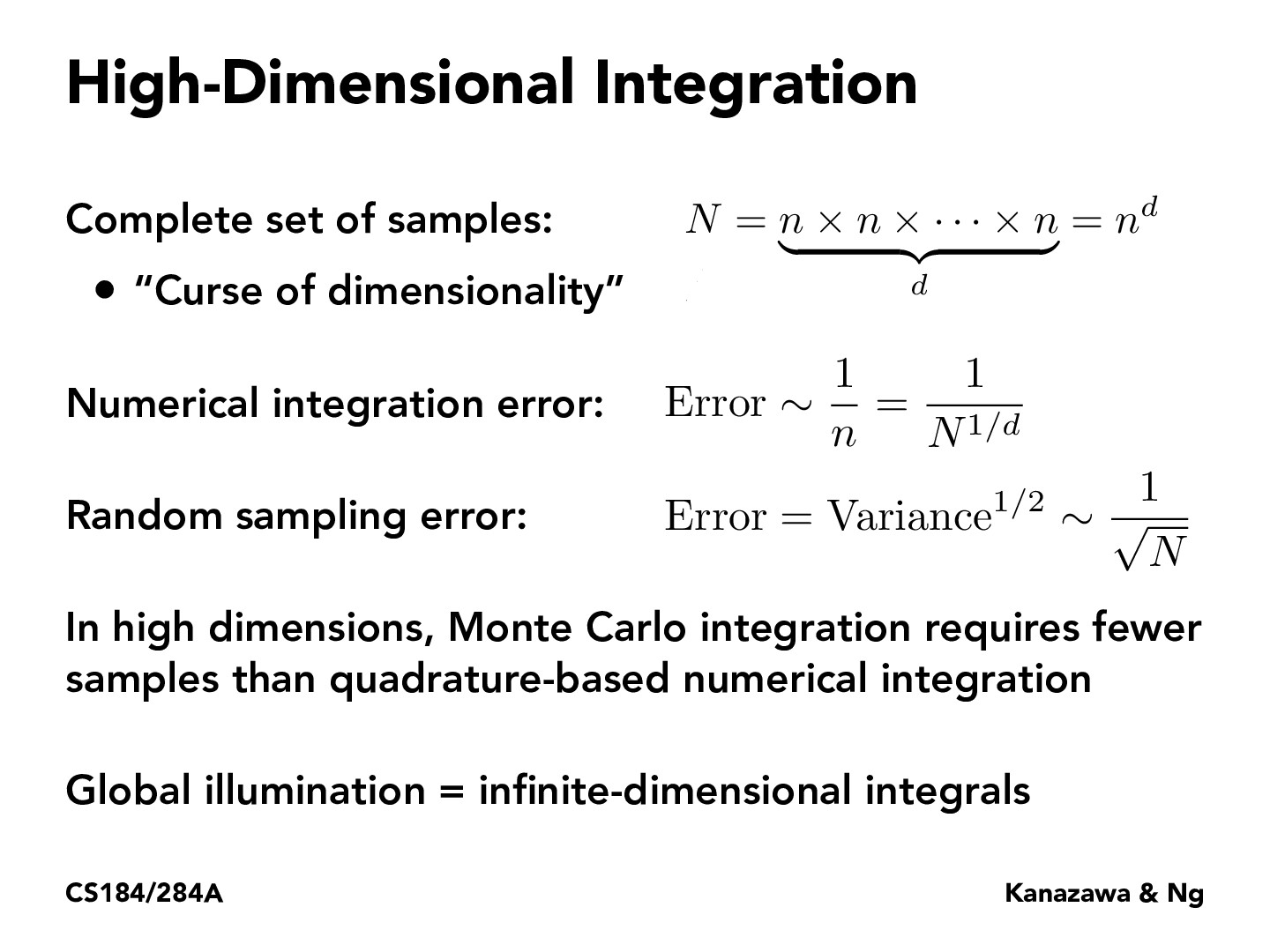Lecture 12: Monte Carlo Integration (9)ronthalanki

I'm was curious why Monte Carlo integration requires fewer samples than quadrature-based numerical integration and found this resource that explains it (https://math.stackexchange.com/questions/425782/why-does-monte-carlo-integration-work-better-than-naive-numerical-integration-in).

TLDR: Monte Carlo samples near areas of large function values whereas Reimann integration requires constant sampling (and to get the same error, you would need a high sampling frequency).selinafeng

I'm having trouble understanding the intuition behind the last bullet point. What would an infinite-dimensional integral mean, and why does global illumination necessarily require it?didvi

In reality, a light ray will bounce throughout the scene an infinite number of times. Determining light in a scene is thus an infinite dimensional integral that we have to estimate.grace858

To clarify, by the two error functions, Monte Carlo integration would require less samples regardless of what the dimension is, as long as the dimension is greater than 2, right? Would they have similar performance given 2 dimensions?j316chuck

They would have similar performance given two dimensions but as the number of dimensions grow monte carlo integration requires less samples because 1/sqrt(N) < 1/N^1/d (numerical integration)

You must be enrolled in the course to comment